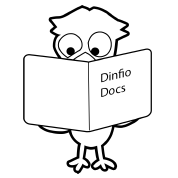### Code Structure

Dinfio Documentation

###### Preface

If you are familiar with C/C++ or JavaScript or other C-style languages, you will easily dive into Dinfio. But curly brackets are not used in Dinfio and you don't need to add semicolon `;` at the end of every statement. Semicolon is only needed when you want to fit multiple code lines into a single line.

``````' This code:

a = 10
b = 20
writeln(a + b)

' Also can be written as:

a = 10; b = 20; writeln(a + b)``````

Dinfio is also not case-sensitive. `a = 10` is equivalent to `A = 10`. `writeln(a)` is also equivalent to `WRITELN(A)`.

###### Code Structure

There are three big blocks in Dinfio code structure: `Import Statements`, `Main Statements`, and `Functions and Classes Declaration`. These blocks must be written in order—cannot be reversed.

``````' Import statements block

import math
import string

' Main statements block

start
a = 23
b = 20
c = do_something(a, b)
d = length("Hello")
stop

' Functions and classes declaration block

function do_something(x, y)
return sqrt(x ^ 2 + y ^ 2)
stop``````

Remember: Pair of keywords `start` and `stop` is optional and just a sweetener. So you can also code:

``````' Import statements block

import math
import string

' Main statements block

a = 23
b = 20
c = do_something(a, b)
d = length("Hello")

' Functions and classes declaration block

function do_something(x, y)
return sqrt(x ^ 2 + y ^ 2)
stop``````

`Import Statements` block and `Functions and Classes Declaration` block are optional, your code can contain only `Main Statements` block.

``````' This code only contains main statements block

start
a = 23
b = 20

writeln(a * b)
stop``````

###### Import Statement

You can import modules using statement `import module_1, [module_2], [module_3], ..., [module_n]`.

``````import math
import string, json

writeln(sin(30))                   ' This function is from module math
writeln(lcase("Hello"))            ' This function is from module string
writeln(json.encode({data: 10}))   ' This function is from module json``````

You can also import other `fio` files using statement `import file_1, [file_2], [file_3], ..., [file_n]` (without `.fio` extension).

``````import other          ' Import file other.fio
import mydir/helper   ' Import file helper.fio in directory mydir

do_something()        ' This function is from file other.fio
writer(some_data)     ' This constant is from file other.fio
do_again(20)          ' This function is from file mydir/helper.fio``````

Here is the content of file `other.fio`. Note: Dinfio only imports functions and classes, all codes that placed outside of functions and classes will not be executed. If you want to declare global variables and function calls, you can place them inside the init function.

``````' Place your init codes inside this init function.
' Function name must be <unique_name>::init, you can use <filename>::init

function other::init()
global some_data = 20
stop

function do_something()
some_data = 40
stop``````

And here is the content of file `mydir/helper.fio`:

``````function do_again(x)
writeln(x ^ 2)
stop``````

Download the example: import-example.zip

 ← A Hello World Programme Index Data Types and Variables →

Documentation version: 1.0.09. Updated on: 17 April 2021.
Dinfio is designed and written by Muhammad Faruq Nuruddinsyah. Copyright © 2014-2021. All rights reserved.